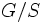# Hypercenter

This article defines a subgroup-defining function, viz., a rule that takes a group and outputs a unique subgroup
View a complete list of subgroup-defining functions OR View a complete list of quotient-defining functions

## Definition

### Symbol-free definition

The hypercenter of a group is defined as the limit of the upper central series of the group.

### Definition with symbols

Let$Z^{\alpha}(G)$ with$\alpha$ varying over the cardinals, denote the upper central series of$G$. Then, there exists a cardinal$\alpha$ at which the upper central series stabilizes. The group$Z^{\alpha}(G)$ for this$\alpha$ is termed the hypercenter.

## Properties

### Reverse monotonicity

The hypercenter subgroup-defining function is reverse monotone. That is, if$H$$G$ are groups, the hypercenter of$H$ contains the itnersection with$H$ of the hypercenter of$G$.

### Idempotence

This subgroup-defining function is idempotent. In other words, applying this twice to a given group has the same effect as applying it once

The hypercenter of the hypercenter is the hypercenter. The image cum fixed-point space for the hypercenter map is the property of being a hypercentral group.

### Quotient-idempotence

This subgroup-defining function is quotient-idempotent: taking the quotient of any group by the subgroup, gives a group where the subgroup-defining function yields the trivial subgroup
View a complete list of such subgroup-defining functions

If$G$ is a group and$S$ is the hypercenter of$G$, then the hypercenter of$G/S$ is trivial.

## Subgroup properties satisfied

The hypercenter of any group is a strictly characteristic subgroup.